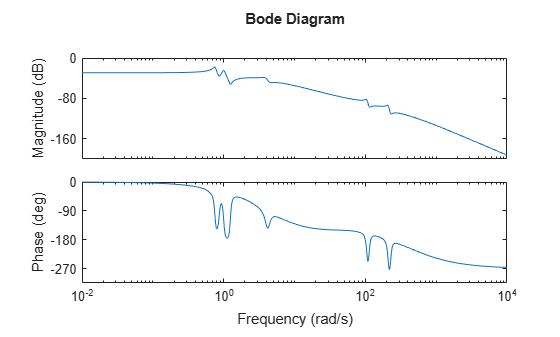# hsvdOptions

(Not recommended) Create option set for computing Hankel singular values and input/output balancing

`hsvdOptions` is not recommended. Use `balredOptions` instead. For more information, see Compatibility Considerations.

## Syntax

```opts = hsvdOptions opts = hsvdOptions(Name,Value) ```

## Description

`opts = hsvdOptions` returns the default options for the `hsvd` and `balreal` commands.

`opts = hsvdOptions(Name,Value)` returns an options set with the options specified by one or more `Name,Value` pair arguments.

## Input Arguments

### Name-Value Arguments

Specify comma-separated pairs of `Name,Value` arguments. `Name` is the argument name and `Value` is the corresponding value. `Name` must appear inside single quotes (```' '```). You can specify several name and value pair arguments in any order as `Name1,Value1,...,NameN,ValueN`.

 `FreqIntervals` Frequency intervals for computing frequency-limited Hankel singular values (`balred`) or balanced realization (`balreal`), specified as a matrix with two columns. Each row specifies a frequency interval ```[fmin fmax]```, where `fmin` and `fmax` are nonnegative frequencies, expressed in the frequency unit of the model. For example:To restrict the computation to the range between 3 rad/s and 15 rad/s, assuming the frequency unit of the model is rad/s, set `FreqIntervals` to `[3 15]`.To restrict the computation to two frequency intervals, 3-15 rad/s and 40-60 rad/s, use `[3 15; 40 60]`.To specify all frequencies below a cutoff frequency `fcut`, use `[0 fcut]`. To specify all frequencies above the cutoff, use ```[fcut Inf]``` in continuous time, or `[fcut pi/Ts]` in discrete time, where `Ts` is the sample time of the model. The default value, `[]`, imposes no frequency limitation and is equivalent to `[0 Inf]` in continuous time or `[0 pi/Ts]` in discrete time. However, if you specify a `TimeIntervals` value other than `[]`, then this limit overrides `FreqIntervals = []`. If you specify both a `TimeIntervals` value and a `FreqIntervals` value, then the computation uses the union of these intervals. Default: `[]` `TimeIntervals` Time intervals for computing time-limited Hankel singular values (`balred`) or balanced realization (`balreal`), specified as a matrix with two columns. Each row specifies a time interval `[tmin tmax]`, where `tmin` and `tmax` are nonnegative times, expressed in the time unit of the model. The software computes state contributions to the system’s impulse response in these time intervals only. For example:To restrict the computation to the range between 3 s and 15 s, assuming the time unit of the model is seconds, set `TimeIntervals` to ```[3 15]```. To restrict the computation to two time intervals, 3-15 s and 40-60 s, use `[3 15; 40 60]`. To specify all times from zero up to a cutoff time `tcut`, use `[0 tcut]`. To specify all times after the cutoff, use `[tcut Inf]`. The default value, `[]`, imposes no time limitation and is equivalent to `[0 Inf]`. However, if you specify a `FreqIntervals` value other than `[]`, then this limit overrides `Timeintervals = []`. If you specify both a `TimeIntervals` value and a `FreqIntervals` value, then the computation uses the union of these intervals. `SepTol` Maximum loss of accuracy value in stable and unstable decomposition. For models with unstable poles, `balred` first extracts the stable dynamics using `stabsep`. Use '`SepTol`' to control the decomposition accuracy. For more information, see `balredOptions`. Default: `SepTol = 'auto'` `Offset` Offset for the stable/unstable boundary. Positive scalar value. In the stable/unstable decomposition, the stable term includes only poles satisfying: `Re(s) < -Offset * max(1,|Im(s)|)` (Continuous time)`|z| < 1 - Offset` (Discrete time) Increase the value of `Offset` to treat poles close to the stability boundary as unstable. Default: `1e-8`

For additional information on the options and how they affect the calculation, see `balredOptions`. The time-limited and frequency-limited state contributions are calculated using the time-limited and frequency-limited controllability and observability Gramians, as described in `gram` and in .

## Examples

collapse all

Compute the Hankel singular values of the system given by:

`$sys=\frac{\left(s+0.5\right)}{\left(s+{10}^{-6}\right)\left(s+2\right)}$`

Use the `Offset` option to force `hsvd` to exclude the pole at $s=1{0}^{-6}$ from the stable term of the stable/unstable decomposition.

```sys = zpk(-.5,[-1e-6 -2],1); opts = hsvdOptions('Offset',.001); hsvd(sys,opts)```
```ans = 2×1 Inf 0.1875 ```

The plot shows that one state is treated as unstable. `hsvd` computes the energy contributions of the stable states only.

Compute the Hankel singular values of a model with low-frequency and high-frequency dynamics. Focus the calculation on the high-frequency modes.

Load the model and examine its frequency response.

```load modeselect Gms bodeplot(Gms)````Gms` has two sets of resonances, one at relatively low frequency and the other at relatively high frequency. Compute the Hankel singular values of the high-frequency modes, excluding the energy contributions to the low-frequency dynamics. To do so, use `hsvdOptions` to specify a frequency interval above 30 rad/s.

```opts = hsvdOptions('FreqInterval',[30 Inf]); hsvd(Gms,opts)```
```ans = 18×1 10-4 × 0.6237 0.4558 0.3183 0.2468 0.0895 0.0847 0.0243 0.0028 0.0000 0.0000 ⋮ ```

## Compatibility Considerations

expand all

Not recommended starting in R2021a

## References

 Gawronski, W. and J.N. Juang. “Model Reduction in Limited Time and Frequency Intervals.” International Journal of Systems Science. Vol. 21, Number 2, 1990, pp. 349–376.